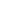Four cars with a mass of 1500 kg each are loaded on a 6 m wide, 12 m long small car ferry. How far, in cm will it sink in the water?

a) 15.2
b) 11.2
c) 10.2
d) 8.3
ISRO Scientist-2010

 8.3. Mass of each car = 1500 kg Gravity is taken as 10 N/s2 Weight = m g Total weight of the cars = 1500 * 4 * 10 (N) Weight of the water displaced = Weight of the car V * ρ * g = 1500 * 4 * 10 Volume of water displaced = (1500*4*10) / (1000*10) V = 6 m3 Volume of water displaced = Volume of car immersed 6 m3 = 6 * 12 * h m3 h = 0.0833 m h = 8.3 cm
Share :
+
Next
« Prev
Prev
Next »